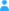Sign in

|

Dependencies in Dataset #365

during TECHNO mission

Entries (observed variables)

Information about observed variables of the current dataset.
No. Name
01 Time_np in sec after midnight (Falcon data system time)
02 IGI body z-axis acceleration [m/s^2]
03 gps2Altitude [m]
04 gps2Latitude [degree (+-90)]
05 gps2Longitude [degree (+-180)]
06 IGI vertical acceleration [m/s^2]
07 IGI actual track angle [degree (0-360)]
08 IGI ground speed [m/s]
09 Event marker Big Button [V]
10 IGI height above WGS84 ellipsoid [m]
11 IGI latitude position above WGS84 [degree (+-90)]
12 IGI longitude position above WGS84 [degree (+-180)]
13 IGI roll angle [degree (+-180)]
14 IGI pitch angle [degree (+-180)]
15 IGI true heading [degree (0-360)]
16 IGI body roll rate [degree/sec]
17 IGI body pitch rate [degree/sec]
18 IGI body yaw rate [degree/sec]
19 IGI body x-axis acceleration [m/s^2]
20 IGI body y-axis acceleration [m/s^2]
21 Angle of Attack [degree (+-180)]
22 Angle of Sideslip [degree (+-180)]
23 IGI east west velocity no schuler correction applied [m/s]
24 Height above Sea Level calculated from met. data (fast) [m]
25 Pressure Altitude [m]
26 Machnumber [Ma]
27 IGI north south velocity no schuler correction applied [m/s]
28 Static Pressure (from NB_PSIA) [hPa]
29 Dynamic Pressure [hPa]
30 Calculated True Airspeed [m/s]
31 Total Air Temperature (deicing corrected, from BDY-TTQ) [K]
32 Potential Temperature [K]
33 Wind Vector East Component [m/s]
34 Wind Vector North Component [m/s]
35 Vertical Velocity (from IRS) [m/s]
36 Wind Vector Vertical Component [m/s]
37 Horizontal Wind Direction [degree (0-360)]
38 Horizontal Windspeed [m/s]
39 Static Air Temperature (from BDY-TTQ) [K]

Dependencies of the observed variables

The interactive table provides a visualization of the dependencies of this dataset's entries (variables) which will hopefully assist you to understand the datasets structure.

Most datasets are simple, e.g. like 10 entries depending on 1 entry. But there are other datasets which are far more complex; with relationships between various entries and even reversed dependencies.

Warning: It works only with JavaScript enabled in your browser.

Direction of relationships

Entry A (=depends on)

Explanation

Enable Javascript and you'll see the dependencies of this dataset explained in this box while hovering over the table on the left.

-->